# JAC Class 9 Maths Solutions Chapter 7 Triangles Ex 7.1

Jharkhand Board JAC Class 9 Maths Solutions Chapter 7 Triangles Ex 7.1 Textbook Exercise Questions and Answers.

## JAC Board Class 9th Maths Solutions Chapter 7 Triangles Ex 7.1

Page-118

Question 1.
In quadrilateral ACBD, AC = AD and AB bisects ∠A (see Fig). Show that ΔABC ≅ ΔABD. What can you say about BC and BD?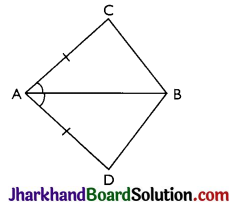Given: AC = AD and AB bisects ∠A
To prove: ΔABC ≅ ΔABD
Proof: In AABC and AABD,
AB = AB (Common)
∠CAB = ∠DAB (AB is bisector)
Therefore, ΔABC ≅ ΔABD by SAS congruence criterion.
BC = BD (By CPCT).Page-119

Question 2.
ABCD is a quadrilateral in which AD = BC and ∠DAB = ∠CBA (see Fig). Prove that
(i) ΔABD ≅ ΔBAC
(ii) BD = AC
(iii) ∠ABD = ∠BAC.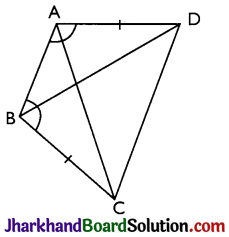Given: AD = BC and ∠DAB = ∠CBA
Proof:
(i) In ΔABD and ΔBAC,
AB = BA (Common)
∠DAB = ∠CBA (Given)
Therefore, ΔABD ≅ ΔBAC by SAS
congruence criterion.

(ii) Since, ΔABD ≅ ΔBAC Therefore, BD = AC (by CPCT)

(iii) Since, ΔABD ≅ ΔBAC Therefore, ∠ABD = ∠BAC (by CPCT)

Question 3.
AD and BC are equal perpendiculars to a line segment AB (see Fig). Show that CD bisects AB.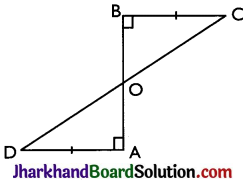Given: AD and BC are perpendiculars to AB.
To prove: CD bisects AB i.e. OA = OB
Proof: In ΔAOD and ΔBOC,
∠A = ∠B = 90° (Perpendicular)
∠AOD = ∠BOC (Vertically opposite angles)
Therefore, ΔAOD ≅ ΔBOC by AAS congruence criterion.
Now, AO = OB (CPCT) i.e. CD bisects AB.

Question 4.
l and m are two parallel lines intersected by another pair of parallel lines p and q (see Fig). Show that ΔABC ≅ ΔCDA.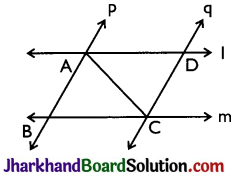Given: l || m and p || q
To prove: ΔABC ≅ ΔCDA
Proof: In ΔABC and ΔCDA,
As l || m
∴ ∠BCA = ∠DAC
(Alternate interior angles) AC = CA (Common)
As p 11q
∴ ∠BAC = ∠DCA (Alternate interior angles)
Therefore, ΔABC ≅ ΔCDA by ASA congruence criterion.Question 5.
Line l is the bisector of an angle ∠A and B is any point on P. BP and BQ are perpendiculars from B to the arms of ∠A (See Fig.) Show that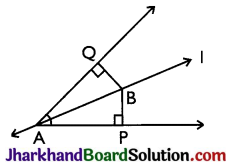(i) ΔAPB ≅ ΔAQB
(ii) BP = BQ or B is equidistant from the arms of ∠A.
Given: l is the bisector of an angle ∠A.
BP and BQ are perpendiculars.
Proof:
(i) In AAPB and AAQB,
∠P = ∠Q (Right angles)
∠BAP = ∠BAQ (l is bisector)
AB = AB (Common)
Therefore, ΔAPB ≅ ΔAQB by AAS congruence criterion.

(ii) BP = BQ by CPCT.
(∵ ΔAPB ≅ ΔAQB)
Therefore, B is equidistant from the arms of ∠A.

Page-120

Question 6.
In Fig, AC = AE, AB = AD and ∠BAD = ∠EAC. Show that BC = DE.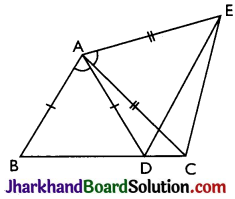To prove: BC = DE
AC = AE (Given)
Therefore, ΔABC ≅ ΔADE by SAS congruence criterion.
BC = DE (by CPCT).

Question 7.
AB is a line segment and P is its mid¬point. D and E are points on the same side of AB such that ∠BAD = ∠ABE and ∠EPA = ∠DPB (see Fig). Show that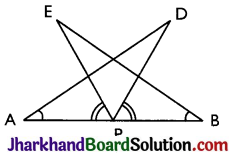(i) ΔDAP ≅ ΔEBP
Given: P is mid-point of AB.
∠BAD = ∠ABE and ∠EPA = ∠DPB
Proof:
(i) ∠EPA = ∠DPB
∠EPA + ∠DPE = ∠DPB + ∠DPE (Adding ∠DPE both sides)
⇒ ∠DPA = ∠EPB
In ΔDAP and ΔEBP,
∠DPA = ∠EPB
AP = BP (P is mid-point of AB)
Therefore, ΔDAP ≅ ΔEBP by ASA congruence criterion.

(ii) AD = BE (by CPCT)Question 8.
In right triangle ABC, right angled at C, M is the mid-point of hypotenuse AB. C is joined to M and produced to a point D such that DM = CM. Point D is joined to point B (see Fig). Show that: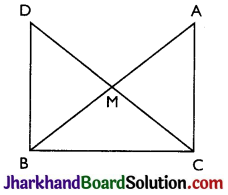(i) ΔAMC ≅ ΔBMD
(ii) ∠DRC is a right angle.
(iii) ΔDBC ≅ ΔACB
(iv) CM = $$\frac{1}{2}$$AB
Given: ∠C = 90°, M is the mid-point of AB and DM = CM
Proof:
(i) In ΔAMC and ΔBMD,
AM = BM (M is the mid-point of AB)
∠CMA = ∠DMB (Vertically opposite angles)
CM = DM (Given)
Therefore, ΔAMC ≅ ΔBMD by SAS congruence criterion.

(ii) ∠ACM = ∠BDM (by CPCT)
Therefore, AC || BD as alternate interior angles are equal.
Now, ∠ACB + ∠DBC = 180° (co-interior angles)
⇒ 90° + ∠DBC = 180°
⇒ ∠DBC – 90°

(iii) In ΔDBC and ΔACB,
BC = CB (Common)
∠ACB = ∠DBC (Right angles)
DB = AC (by CPCT)
Therefore, ΔDBC ≅ ΔACB by SAS congruence criterion.

(iv) DC = AB (CPCT)
DM + CM = AB
⇒ CM + CM = AB (∵ DM = CM)
⇒ CM = AB
⇒ CM = $$\frac{1}{2}$$ AB